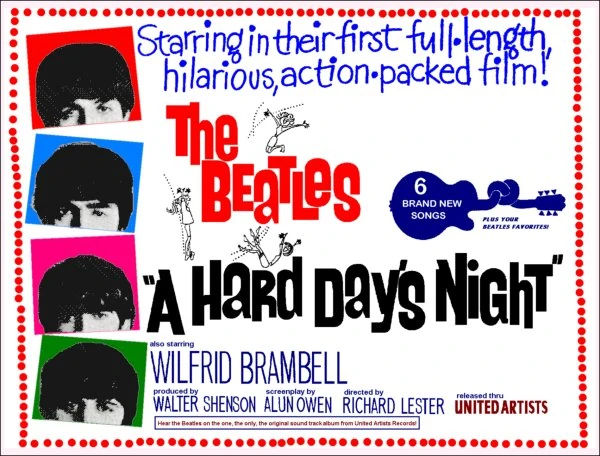# The Beatles meet Mathematics & PhysicsMathematics, Physics and A Hard Day’s Night

In this article we shall use mathematics and the physics of sound to unravel one ofthe mysteries of rock ’n’ roll – how did the Beatles play the opening chord of A Hard Day’s Night? The song may never sound the same to you again.

I just love this paper.  Professor Jason Brown sampled the famous opening chord of this song and (using Math) separated out all the distinct frequencies in the clip.  From this, he was able to determine each individual note that was played.  From there, he determined exactly what each member of the band played.  He even discovered a surprising element relating to George Martin’s 5th Beatle status.

Professor Brown took each frequency and converted it to a musical note on the Western scale.  Here is the function he used to do this:$f(x) = 12 log_2(\frac{x}{220})$    (…where 220 hertz is the frequency for A natural.)

I thought I’d try his calculation myself, because it’s a good opportunity to use the change of base formula for logarithms.  If you look in the original white paper, the first frequency in the table is 110.34   Let’s plug this into the function:$f(110.34) = 12 log_2(\frac{110.34}{220}) = 12 log_2(.5015)$

How do you evaluate the above expression?  There is no$log_2()$ button on most calculators.  Well, here’s where the change of base formula for logs comes in:$log_b(x) = \frac{log_d(x)}{log_d(b)}$   So, let’s choose$log_{10}$ (since calculators do have this) and continue:$12 log_2(.5015)=12(\frac{log_{10}.5015}{log_{10}2}) = 12(-.9957) = -11.9466$

In other words, 110.34hz is -11.9466 semi-tones below the note of A.  It should really be 12, but as Professor Brown noted, the Beatles’ instruments weren’t in perfect tune, so the values are not whole numbers!So, which note is 12 semi-tones below A?  Actually, 12 semi-tones makes an octave, so the answer an A note.  Using this method, he determined every note that was played.  The rest of the paper describes how he deduced which groups of notes were played by which instrument/band member.  Fascinating.